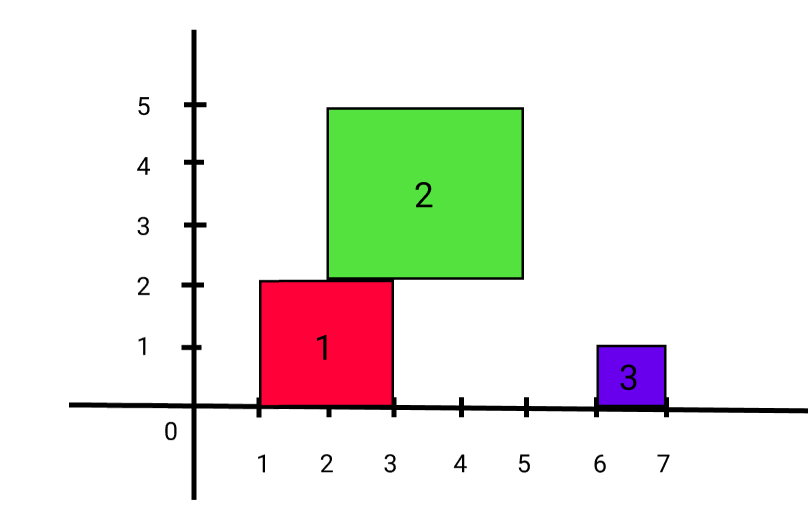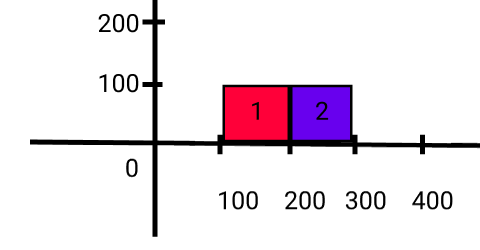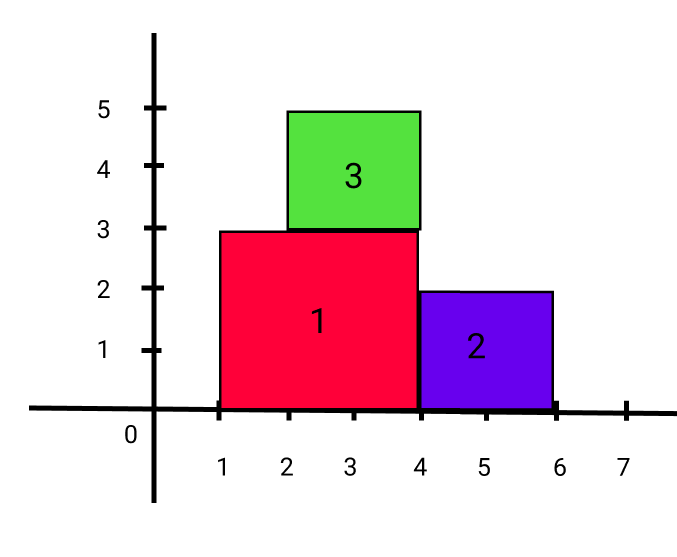Problem title
Difficulty
Avg time to solve

Count of Smaller Elements
Hard
45 mins
Sequence Reconstruction
Moderate
30 mins
The Earliest Moment When Everyone Become Friends
Hard
45 mins
Ninja and Meteorites
Moderate
25 mins
Split BST
Moderate
25 mins
Print array Sum
Easy
--
Invert a Binary Tree
Moderate
45 mins
Verify Preorder Sequence in Binary Search Tree
Moderate
30 mins
Validate Binary Tree Nodes
Moderate
15 mins
Delete Nodes And Return Forest
Moderate
20 mins4

# Ninja and Meteorites

Difficulty: MEDIUM
Avg. time to solve
25 min
Success Rate
75%

Problem Statement

#### For example:

``````Let ‘N’ = 3 and ‘METEORITES’ = [[1, 2], [2, 3], [6, 1]].
The position and size of each meteorite are: </br>
‘Meteorite1’:  At 1 position and size of 2.
‘Meteorite2’:  At 2 position and size of 3.
‘Meteorite3’:  At 6 position and size of 1.
````````````So when the first meteorite falls maximum height till now is 2.
When the second meteorite falls maximum height till now is 5.
When the third meteorite falls maximum height till now is 5.
``````
##### Input Format
``````The first line of input contains an integer ‘T’ which denotes the number of test cases or queries to be run. Then the test cases follow.

The first line of each test case contains an integer ‘N’ representing the number of meteorites.

The next ‘N’ line of each test case contains two single space-separated integers ‘X’ and ‘SIZE’ representing the leftmost X-coordinate and size of the meteorites respectively.
``````
##### Output Format :
``````For each test case, print a single line containing the current highest height of any meteorite after each meteorite falls from space.

The output of each test case will be printed in a separate line.
``````

#### Note:

``````You do not need to print anything; it has already been taken care of. Just implement the given function.
``````
##### Constraints:
``````1 <= ‘T’ <= 10
1 <= ‘N’ <= 1000
1 <= ‘X’ <= 100000
1 <= ‘SIZE’ <= 10000

Where ‘T’ denotes the total number of test cases, ‘N’ represents the number of meteorites,’ X’ denotes the leftmost X-coordinate of the current meteorite and ‘SIZE’ represents the size of the current meteorite.

Time Limit: 1 second
``````
##### Sample Input 1:
``````1
2
100 100
200 100
``````
##### Sample Output 1:
``````100 100
``````

#### Explanation for Sample Output 1:

``````For sample test case 1:
````````````So, when the first meteorite falls maximum height till now is 100.
When the second meteorite falls maximum height till now is 100.
``````
##### Sample Input 2:
``````1
3
1 3
4 2
2 2
``````
##### Sample Output 2:
``````3 3 5
``````

#### Explanation for Sample Output 2:

``````For sample test case 1:
````````````So when the first meteorite falls maximum height till now is 3.
When the second meteorite falls maximum height till now is 3.
When the third meteorite falls maximum height till now is 5.
``````Console